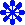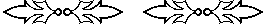Math 105   History of Mathematics
Course Overview

Prof. D Joyce
Department of Mathematics and Computer Science
Clark University

What are we going to study this semester? Why, the history of mathematics, of course! But what does that encompass? Too much for a semester, for one thing. Okay, this isn't the whole of the history of mathematics; it's more of an introduction. In fact, we won't get to anything recent.

So, where do we begin, and how far do we get? Well, what's supposed to be included in mathematics, anyway? Do we include arithmetic? How about counting? Counting, and maybe arithmetic, began before history. What I mean by that is that people probably counted, and maybe added and subtracted, before they could write. We have time to look at at little bit of this prehistory. We'll look at Denise Schmandt-Besserat's research into tokens of preliterate Mesopotamia, ranging from 8000 B.C.E. to 3000 B.C.E. Unfortunately, that's one thing Victor J. Katz leaves out of his text, A History of Mathematics, the book we're using for our course text, so I'll have to introduce that subject myself. Overall, I think his book is an excellent text.

Counting can barely be considered mathematics, so we'll move quickly on to historic times, where mathematics really began its development. We'll look at the mathematics of Egypt and literate Mesopotamia, in particular. We'll see how they denoted numbers, their arithmetic, how they solved what we call linear equations and other equations, and some geometry. This material is not so primitive as you might expect at such an early time (ca. 2000 B.C.E.). Both cultures understood a bit of geometry and number theory. The Babylonians, for instance, knew the so-called Pythagorean theorem. (Perhaps "rule of the right triangle" would be a better term since (1) we're speaking of a time 1300 years before Pythagoras, and (2) the word "theorem" suggests a proof was known, and there's no indications that the Babylonians had a proof or even recognized the need for a proof.) That's all in chapter 1. We can't spend a long time on it, but you can already see that the subject we're considering is pretty big.

The next thing we'll look at is Greek mathematics. You could spend the rest of your life on it, but we'll condense it into a month. In 300 years, from the time of Thales (ca. 600 B.C.E.) to the time of Euclid (ca. 300 B.C.E.), mathematics changed from an empirical subject to a theoretical one. How did that happen? That's a big question, and, because so few original (or even secondary) sources exist, we can't expect to answer it satisfactorily. But we can look at some of that mathematics, especially Euclid's. Truly marvelous stuff. It's fully developed mathematics, in the modern sense of the word "mathematics." Most of it is very interesting geometry, but there's a fair amount of number theory and some very abstract algebra (Eudoxus' theory of proportion). We'll spend quite a bit of time on chapter 2.

After Euclid, the Greeks ("Hellenistic culture" might be a better term, since much of it occurs outside Greece, but all in the Greek language) developed more mathematics ranging from astronomy to algebra, and we'll look at that in the next three chapters. Someplace I've got to cut back, or we'd never reach where I want to go, namely, to the beginnings of calculus, and I'm afraid these are the chapters that we'll have to squeeze. The subjects are interesting, but the subjects are a little more advanced, and that gives me an excuse to rush ahead. (Actually, a course that emphasized this period and ended at 500 C.E. would be a good one, but only if there were more courses afterwards that could do the last millennium and a half.)

Islamic culture took up the mathematics of the Hellenistic culture after the latter waned. We'll look at that in chapter 9, and the mathematics of the older cultures of China and India in chapters 7 and 8. For the most part, Chinese and Indian mathematics continued with a nontheoretical attitude, but their mathematics was significant nonetheless. Except through their influence on Islamic mathematics, the mathematics of China and India has not influenced modern mathematics.

Europe, excepting Greece and its colonies, was virtually mathematics-free until some Greek and Islamic mathematics was translated from Arabic into Latin in the 13th century. Exciting new developments began with the Scholastics in the 14th century that eventually led to calculus in the 17th century. We'll spend quite a bit of time on the mathematical Renaissance in Europe. There were so many new subjects developed in those three or four centuries: algebra, symbolic algebra, logarithms, analytic geometry, number theory, probability, projective geometry, and eventually, the concepts underlying calculus. The actual calculus, that is, the rules to manipulate symbolic expressions to find derivatives and integrals, had to wait until Newton and Leibniz in the 17th century.Although the course stops there, I don't want to give the impression that mathematics died in 1700. Since then every decade has produced more mathematics than the previous decade. It's just that the mathematics gets more advanced (that is, it depends on more previous mathematics) and more abstract, and we don't have time to look at it in just one semester. Frankly, I'd like to see every mathematics course include some of the history of its subject, and that's just what I try to do in the other courses I teach.